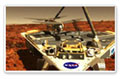Synopsis: To fly around on the surface of Mars, an aircraft would need to travel at speeds of over 250 MPH just to stay aloft in the very thin atmosphere. Take off and landing on the rocky terrain to gather samples would be nearly impossible. What is the solution?
Running Time: 02:36 minutes

Critical Thinking:

If you want to build a vehicle that will fly on Mars, you might start by asking two questions:

1) How do things fly on Earth?

2) How is Mars different from Earth?

## Attack the problem

1. If you think about all of the things that can move through the air on Earth, you soon realize that there are actually many methods of flight. Use these resources to find out more about some of them:

Birds and Insects

Blimps

2. Mars is about half the size of Earth. The gravitational force (F) on a body of mass (m) on the surface of a planet of mass (M) and radius (R) is given by Newton’s equation F = GMm/R2. If you plug in the fact that Mars has about half the radius of Earth and, therefore, roughly 1/8th of the volume, you will discover that if Mars had the same composition as Earth, its gravity would be about 50% of ours. (In fact, its gravity is a little less than 40% of Earth’s–the fact that it’s lower than expected based on its size tells you that the interior of Mars does not have the same composition as that of the earth.)

Lower gravity on the surface means a lower “escape velocity” is required for air molecules to move out of the atmosphere and into space; it’s easier for the air to dissipate into space, hence a thinner atmosphere. In fact, the density of air on Mars is about 1/100th of the density on Earth—but is that difference all due to the lower gravity, or might there be other factors at play?

Explore this topic further with this resource:

Escape Velocity